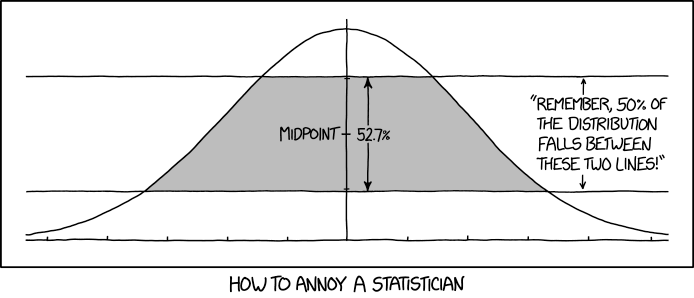# Normal horizontiles

From XKCD todayHow can we check this calculation?

First, we need to know where the lines are on the y-axis. They are separated by 52.7% of the height of the distribution, and looks as if they are meant to exclude the same height above and below. We don’t need to worry about the mean of the distribution (obviously), or the scale (less obviously). The reason the scale is not needed is that rescaling the x axis shrinks all three of the areas under the curve by the same factor, and since they add up to 1, they stay the same. If we write $$p$$ and $$q$$ for the two heights, we have

$\begin{eqnarray*} p-q &=& \phi(0)\times 0.572\\ p+q&=&\phi(0) \end{eqnarray*}$ from which we get $q = \phi(0)\times (1-0.527)/2$ or in R

q<- dnorm(0)*(1-0.527)/2
p<- dnorm(0)-q

Let $$-a$$ and $$a$$ be the x-axis values for the lower ends of the filled strip and $$-b$$ and $$b$$ be the corresponding upper ends. The area we want can be worked out at least three ways.

First, take the area above the lower line and subtract the area above the upper line $\int_{-a}^a (\phi(x)-q)\,dx-\int_{-b}^b (\phi(x)-p)\,dx$

Second, define a function that’s the height of the coloured strip at $$x$$: it’s the maximum of (the minimum of $$\phi(x)-q$$ and 0) and $$p-q$$: $\int_{-a}^a ((\phi(x)-q)\vee 0)\wedge (p-q)\,dx$

Third, take out the rectangle in the middle of the strip, which is $$2b$$ wide and $$p-q$$ high, and then use integration on the curvy bits on each side: $\int_{-a}^{-b} \phi(x)-q\,dx+ 2b(p-q)+\int_b^a \phi(x)-q\,dx$

In R, first we need to solve $$\phi(x)=q$$ and $$\phi(x)=p$$, for which we need a bound on $$p$$ and $$q$$; I’m using 5, which is clearly enough, since $$\phi(5)=10^{-\textrm{substantial}}$$

a<- uniroot(function(x) dnorm(x)-q, lower=0, upper=5)$root b<- uniroot(function(x) dnorm(x)-p, lower=0, upper=5)$root

Now do the integration three ways

integrate( function(x) dnorm(x)-q,lower=-a, upper=a)$value- integrate( function(x) dnorm(x)-p,lower=-b, upper=b)$value
##  0.5001705
integrate(function(x) dnorm(x)-q,lower=-a, upper=-b)$value+ 2*b*(p-q)+ integrate(function(x) dnorm(x)-q,lower=b, upper=a)$value
##  0.5001705
integrate(function(x) pmin(pmax(dnorm(x)-q,0),p-q),lower=-a, upper=a)\$value
##  0.5001658

And it works[Snipe by slobirdr on Flickr]

Update: As Peter Dalgaard points out, the integrals can be replaced with calls to pnorm, since the integrand is just $$\phi(x)$$ and constants. For example, $\int_{-a}^{-b} \phi(x)-q\,dx+ 2b(p-q)+\int_b^a \phi(x)-q\,dx$ gives

(pnorm(-b)-pnorm(-a))-q*(-b - -a)+2*b*(p-q)+(pnorm(a)-pnorm(b))-q*(a-b)
##  0.5001705

Also, if you happen to know the formula $\phi(x)=\frac{1}{\sqrt{2\pi}}e^{-x^2/2}$, aka dnorm(x), you could compute $$a$$ and $$b$$ directly $\phi^{-1}(d)= \sqrt{-2\log(d\sqrt{2\pi})}$

argdnorm<-function(d) sqrt(-2*log(d*sqrt(2*pi)))
a
##  1.698121
argdnorm(q)
##  1.698121
b
##  0.7346321
argdnorm(p)
##  0.7346321

I think it’s worth not doing this: while it happens to be the case that $$\phi(x)$$ has a closed-form inverse, there isn’t any good reason I know of that it should have one, and I’m happy to invert dnorm numerically.